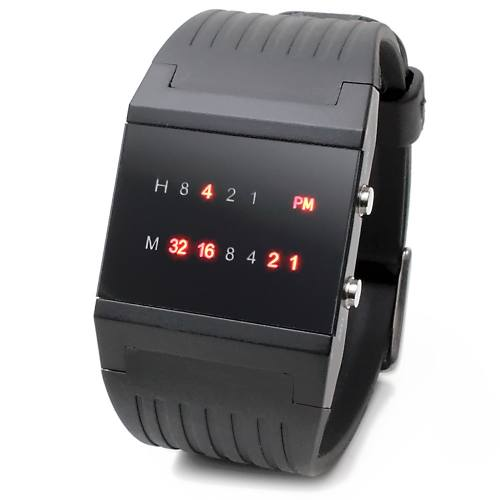## Algorithm

Problem Name: 401. Binary Watch

A binary watch has 4 LEDs on the top to represent the hours (0-11), and 6 LEDs on the bottom to represent the minutes (0-59). Each LED represents a zero or one, with the least significant bit on the right.

• For example, the below binary watch reads `"4:51"`.Given an integer `turnedOn` which represents the number of LEDs that are currently on (ignoring the PM), return all possible times the watch could represent. You may return the answer in any order.

The hour must not contain a leading zero.

• For example, `"01:00"` is not valid. It should be `"1:00"`.

The minute must be consist of two digits and may contain a leading zero.

• For example, `"10:2"` is not valid. It should be `"10:02"`.

Example 1:

```Input: turnedOn = 1
Output: ["0:01","0:02","0:04","0:08","0:16","0:32","1:00","2:00","4:00","8:00"]
```

Example 2:

```Input: turnedOn = 9
Output: []
```

Constraints:

• `0 <= turnedOn <= 10`

## Code Examples

### #1 Code Example with C Programming

```Code - C Programming```

``````
#define MAX_HOUR 12  // this should be 13 so it includes 12:xx pm.
#define MAX_MIN  60
​
char** readBinaryWatch(int num, int* returnSize) {
char **pp, *p;
int sz = MAX_HOUR * MAX_MIN;
​
int hh; // number of digits in hours
int mm; // number of digits in minutes
int i, j, k, x;

i = 0;
while (i < MAX_HOUR) {
hh[i] = 0;
k = i;
while (k != 0) {
while (!(k & 0x1)) k = k >> 1;
k = k >> 1;
hh[i] ++;
}
i ++;
}
i = 0;
while (i < MAX_MIN) {
mm[i] = 0;
k = i;
while (k != 0) {
while (!(k & 0x1)) k = k >> 1;
k = k >> 1;
mm[i] ++;
}
i ++;
}

*returnSize = 0;

pp = malloc(sz * sizeof(char *));
p = malloc(sz * 6 * sizeof(char));
if (!pp || !p) return NULL;

k = 0;
i = 0;
while (i < MAX_HOUR) {
j = 0;
while (j < MAX_MIN) {
if (hh[i] + mm[j] == num) {
pp[k ++] = p;
sprintf(p, "%d:%02d", i, j);
p += 6;
}
j ++;
}
i ++;
}
*returnSize = k;

return pp;
}
``````
Copy The Code &

Input

cmd
turnedOn = 1

Output

cmd
["0:01","0:02","0:04","0:08","0:16","0:32","1:00","2:00","4:00","8:00"]

### #2 Code Example with Java Programming

```Code - Java Programming```

``````
class Solution {
return IntStream.range(0, 12)
.boxed()
.flatMap(
h -> IntStream.range(0, 60)
.boxed()
.filter(s -> Integer.bitCount(h) + Integer.bitCount(s) == turnedOn)
.map(m -> String.format("%d:%02d", h, m))
)
.collect(Collectors.toList());
}
}
``````
Copy The Code &

Input

cmd
turnedOn = 1

Output

cmd
["0:01","0:02","0:04","0:08","0:16","0:32","1:00","2:00","4:00","8:00"]

### #3 Code Example with Javascript Programming

```Code - Javascript Programming```

``````
const output = []
for (let h = 0; h < 12; h++) {
for (let m = 0; m < 60; m++) {
const ones = Number(h * 64 + m)
.toString(2)
.split('')
.filter(d => d === '1').length
if (ones === num) output.push(m < 10 ? `\${h}:0\${m}` : `\${h}:\${m}`)
}
}
return output
}
``````
Copy The Code &

Input

cmd
turnedOn = 9

Output

cmd
[]

### #4 Code Example with Python Programming

```Code - Python Programming```

``````
class Solution:
"""
:type num: int
:rtype: List[str]
"""
return ['%d:%02d' % (h, m)
for h in range(12) for m in range(60)
if (bin(h) + bin(m)).count('1') == num]
``````
Copy The Code &

Input

cmd
turnedOn = 9

Output

cmd
[]

### #5 Code Example with C# Programming

```Code - C# Programming```

``````
using System.Collections.Generic;

namespace LeetCode
{
public class _0401_BinaryWatch
{
{
var results = new List();

for (int i = 0; i < 720; i++)
{
int hour = i / 60;
int minute = i % 60;

if (BitCount(hour) + BitCount(minute) == num)
}

return results;
}

private static int BitCount(int num)
{
var result = 0;
while (num > 0)
{
result++;
num &= num - 1;
}

return result;
}
}
}
``````
Copy The Code &

Input

cmd
turnedOn = 9

Output

cmd
[]